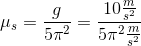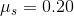# MCAT Physical : Period, Wavelength, and Frequency

## Example Questions

### Example Question #1 : Period, Wavelength, And Frequency

An amusement ride is used to teach students about centripetal force. The ride is a circlular wall that you place your back on. The wall and floor then begin to spin. Once it reaches a certain rotational velocity, the floor drops, and the students are pinned to the wall as a result of centripital force.

The diameter of the ride is 10m. The minimum frequency of rotation that results in students being pinned to the wall after the floor drops is 0.5Hz. What is the coefficient of static friction between the students and the wall?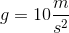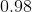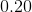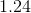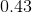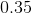Explanation:

There is quite a lot going on in this problem. However, we will take it one step at a time so that you can understand the logical progression of thought it takes to solve this problem.

The first major step will be converting the frequency into a centripetal acceleration.

Convert the frequency into a velocity: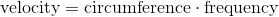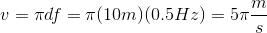This is how fast the outer walls of the ride are traveling. We can convert this into centripetal acceleraton using the expression: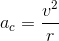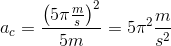Then, we can create an expression for centripetal force for a student with mass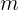: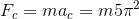Now that we have a term for the centirpetal force, we can work toward finding the force of friction. The centripetal force is also the normal force used in calculating frictional force. If the student is pinned to the wall, then the frictional force is exactly equal to the student's weight.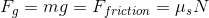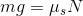Plugging in our expression for the normal force: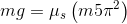Cancel out mass and rearrange for the coefficient of friction: